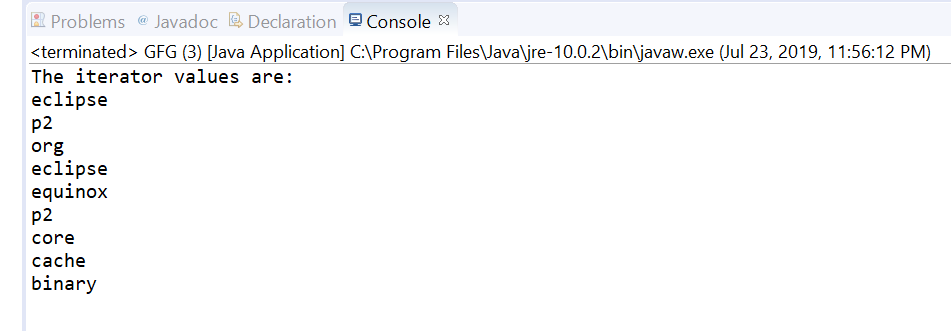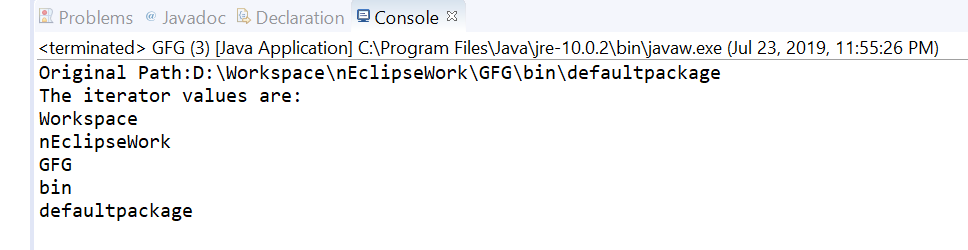# Path iterator() method in Java with Examples

The iterator() method of java.nio.file.Path used to returns an iterator of the name elements which construct this path.
The first element of this iterator contains the name element that is closest to the root in the directory hierarchy, the second element is the next closest, and so on. The last element of this iterator is the name of the file or directory denoted by this path. The root component is not returned by the iterator.

Syntax:

```default Iterator<Path> iterator()
```

Parameters: This method accepts nothing.It is parameter less method.

Return value: This method returns an iterator over the name elements of this path.

Below programs illustrate iterator() method:
Program 1:

 `// Java program to demonstrate ` `// java.nio.file.Path.iterator() method ` ` `  `import` `java.nio.file.Path; ` `import` `java.nio.file.Paths; ` `import` `java.util.*; ` ` `  `public` `class` `GFG { ` `    ``public` `static` `void` `main(String[] args) ` `    ``{ ` ` `  `        ``// create object of Path ` `        ``Path path ` `            ``= Paths.get(``"D:\\eclipse\\p2"` `                        ``+ ``"\\org\\eclipse\\equinox\\p2\\core"` `                        ``+ ``"\\cache\\binary"``); ` ` `  `        ``// Creating an iterator ` `        ``Iterator elements ` `            ``= path.iterator(); ` ` `  `        ``// Displaying the values ` `        ``System.out.println(``"The iterator values are: "``); ` `        ``while` `(elements.hasNext()) { ` `            ``System.out.println(elements.next()); ` `        ``} ` `    ``} ` `} `

Output:Program 2:

 `// Java program to demonstrate ` `// java.nio.file.Path.iterator() method ` ` `  `import` `java.nio.file.Path; ` `import` `java.nio.file.Paths; ` `import` `java.util.*; ` ` `  `public` `class` `GFG { ` `    ``public` `static` `void` `main(String[] args) ` `    ``{ ` ` `  `        ``// create object of Path ` `        ``Path path ` `            ``= Paths.get(``"D:\\Workspace"` `                        ``+ ``"\\nEclipseWork"` `                        ``+ ``"\\GFG\\bin\\defaultpackage"``); ` ` `  `        ``System.out.println(``"Original Path:"` `                           ``+ path); ` ` `  `        ``// Creating an iterator ` `        ``Iterator elements ` `            ``= path.iterator(); ` ` `  `        ``// Displaying the values ` `        ``System.out.println(``"The iterator values are: "``); ` `        ``while` `(elements.hasNext()) { ` `            ``System.out.println(elements.next()); ` `        ``} ` `    ``} ` `} `

Output:Whether you're preparing for your first job interview or aiming to upskill in this ever-evolving tech landscape, GeeksforGeeks Courses are your key to success. We provide top-quality content at affordable prices, all geared towards accelerating your growth in a time-bound manner. Join the millions we've already empowered, and we're here to do the same for you. Don't miss out - check it out now!